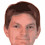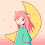## Thursday, 24 March 2011

### RGB/HSV in HLSL 3

DDRKirby(ISQ) had problems with the HLSL code for "RGBtoHSV" in my original posting on this subject. I've had a chance to look at this in more detail and believe I know what the problem is/was. Some versions of the HLSL compiler have difficulty generating correct code for component swizzling under very specific circumstances. A minor refactoring seems to solve the problem:

float3 Hue(float H)
{
float R = abs(H * 6 - 3) - 1;
float G = 2 - abs(H * 6 - 2);
float B = 2 - abs(H * 6 - 4);
return saturate(float3(R,G,B));
}

float3 HSVtoRGB(in float3 HSV)
{
return ((Hue(HSV.x) - 1) * HSV.y + 1) * HSV.z;
}

float3 RGBtoHSV(in float3 RGB)
{
float3 HSV = 0;
#if NO_ASM
HSV.z = max(RGB.r, max(RGB.g, RGB.b));
float M = min(RGB.r, min(RGB.g, RGB.b));
float C = HSV.z - M;
#else
float4 RGB4 = RGB.rgbr;
asm { max4 HSV.z, RGB4 };
asm { max4 RGB4.w, -RGB4 };
float C = HSV.z + RGB4.w;
#endif
if (C != 0)
{
float4 RGB0 = float4(RGB, 0);
float4 Delta = (HSV.z - RGB0) / C;
Delta.rgb -= Delta.brg;
Delta.rgb += float3(2,4,6);
Delta.brg = step(HSV.z, RGB) * Delta.brg;
#if NO_ASM
HSV.x = max(Delta.r, max(Delta.g, Delta.b));
#else
float4 Delta4 = Delta.rgbr;
asm { max4 HSV.x, Delta4 };
#endif
HSV.x = frac(HSV.x / 6);
HSV.y = 1 / Delta.w;
}
return HSV;
}

The major change (which produces the same optimized code as I expected from the original version) is highlighted. I'm not saying this will fix everyone's woes, but it certainly corrects the problem with the one situation I came across.

1.thanks for sharing this great code. i especially like the simplicity of the HSVtoRGB() function. any chance you could provide a HSLtoRGB() function aswell? the functions i found so far were slow, complicated and/or inaccurate.

2.This comment has been removed by the author.

3.Sorry, Anon. I actually re-read your query, instead of making false assumptions. I guess HSL variants shouldn't be too difficult. I'll have a think about it.

4.Anon, it's now available at http://www.chilliant.com/rgb2hsv.html
Hope this helps.

5.thank you so much!

6.I'm just now catching up on this (years later) after realizing that my HSV/RGB shaders need some optimization as they perform pretty poorly on certain GPU targets. I appreciate the follow through and am glad that I could help in a minor way along the journey. I'm off to read through the rest of the posts as well now ;)